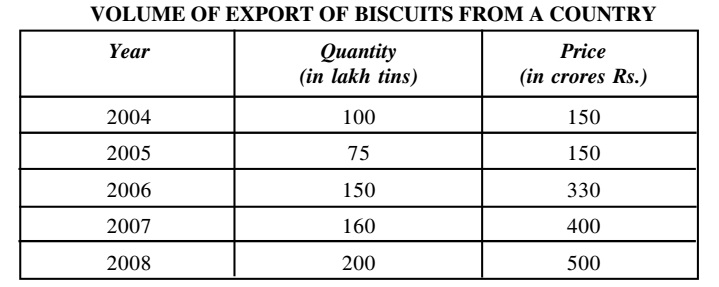## Table chart

#### Data Interpretation

Direction: Figures of biscuit exports from a country during various years are given in the following table. Study the table carefully and answer the questions that follows:1. During which year per tin export price remained at lowest level?

1. Export price per tin in 2004 = Price in 2004/ Quantity in 2004
Export price per tin in 2005 = Price in 2005 / Quantity in 2005
Export price per tin in 2006 = Price in 2006 / Quantity in 2006
Export price per tin in 2007 = Price in 2007/ Quantity in 2007
Export price per tin in 2008 = Price in 2008/ Quantity in 2008

##### Correct Option: A

Export price per tin in 2004 = Price in 2004/ Quantity in 2004
Export price per tin in 2004 = 150 crores/ 100 lakhs = Rs. 150
Export price per tin in 2005 = Price in 2005 / Quantity in 2005
Export price per tin in 2005 = Rs. 150 crores/Rs. 75 lakhs = Rs. 200
Export price per tin in 2006 = Price in 2006 / Quantity in 2006
Export price per tin in 2006 = Rs. 330 crores/Rs. 150 lakhs = Rs. 220
Export price per tin in 2007 = Price in 2007/ Quantity in 2007
Export price per tin in 2007 = Rs. 400 crores/Rs. 160 lakhs = Rs. 250
Export price per tin in 2008 = Price in 2008/ Quantity in 2008
Export price per tin in 2008 = Rs. 500 crores/Rs. 200 lakhs = Rs. 250

1. During which years price per tin remained at the same level?

1. Export price per tin in 2004 = Price in 2004/ Quantity in 2004
Export price per tin in 2005 = Price in 2005 / Quantity in 2005
Export price per tin in 2006 = Price in 2006 / Quantity in 2006
Export price per tin in 2007 = Price in 2007/ Quantity in 2007
Export price per tin in 2008 = Price in 2008/ Quantity in 2008

##### Correct Option: C

Export price per tin in 2004 = Price in 2004/ Quantity in 2004
Export price per tin in 2004 = 150 crores/ 100 lakhs = Rs. 150
Export price per tin in 2005 = Price in 2005 / Quantity in 2005
Export price per tin in 2005 = Rs. 150 crores/Rs. 75 lakhs = Rs. 200
Export price per tin in 2006 = Price in 2006 / Quantity in 2006
Export price per tin in 2006 = Rs. 330 crores/Rs. 150 lakhs = Rs. 220
Export price per tin in 2007 = Price in 2007/ Quantity in 2007
Export price per tin in 2007 = Rs. 400 crores/Rs. 160 lakhs = Rs. 250
Export price per tin in 2008 = Price in 2008/ Quantity in 2008
Export price per tin in 2008 = Rs. 500 crores/Rs. 200 lakhs = Rs. 250
it is clear that per tin export price was same in 2007 and 2008.

1. The export price of biscuit increased from 2004 to 2008 by how much percent?

1. From the level of 2004 percentage increase in export price in 2008 = (Price in 2008 - price in 2004) x 100/price in 2004

##### Correct Option: C

From the level of 2004 percentage increase in export price in 2008 = (Price in 2008 - price in 2004) x 100/price in 2004
From the level of 2004 percentage increase in export price in 2008 = (500 - 150) x 100/150 = 2331/3%

1. The difference between the number of biscuit tins exported in 2008 and that in 2007 is :

1. Required difference = (Quantity in 2008 - Quantity in 2007)

##### Correct Option: D

Required difference = (200 - 160) = 40 lakhs tins = 40,00,000 tins.

1. During which year per tin export price remained at Highest level?

1. Export price per tin in 2004 = Price in 2004/ Quantity in 2004
Export price per tin in 2005 = Price in 2005 / Quantity in 2005
Export price per tin in 2006 = Price in 2006 / Quantity in 2006
Export price per tin in 2007 = Price in 2007/ Quantity in 2007
Export price per tin in 2008 = Price in 2008/ Quantity in 2008

##### Correct Option: A

Export price per tin in 2004 = Price in 2004/ Quantity in 2004
Export price per tin in 2004 = Rs. 150 crores/ Rs. 100 lakhs = Rs. 150
Export price per tin in 2005 = Price in 2005 / Quantity in 2005
Export price per tin in 2005 = Rs. 150 crores/Rs. 75 lakhs = Rs. 200
Export price per tin in 2006 = Price in 2006 / Quantity in 2006
Export price per tin in 2006 = Rs. 330 crores/Rs. 150 lakhs = Rs. 220
Export price per tin in 2007 = Price in 2007/ Quantity in 2007
Export price per tin in 2007 = Rs. 400 crores/Rs. 160 lakhs = Rs. 250
Export price per tin in 2008 = Price in 2008/ Quantity in 2008
Export price per tin in 2008 = Rs. 500 crores/Rs. 200 lakhs = Rs. 250
it is clear that per tin export price was highest in 2007 and 2008.